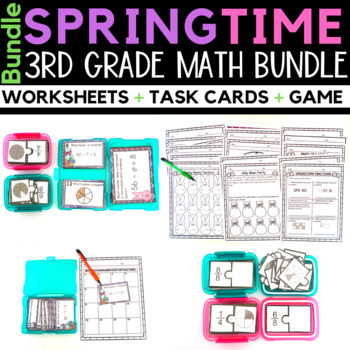Spring Math Worksheets and Centers Bundle3rd - 4th, Homeschool
Subjects
Standards
Resource Type
Formats Included
• Zip
\$12.00
Bundle
List Price:
\$15.00
You Save:
\$3.00
\$12.00
Bundle
List Price:
\$15.00
You Save:
\$3.00

Also included in

1. Worksheets, games, and centers for every season and holiday!
\$50.00
\$69.00
Save \$19.00

Description

This spring worksheets for third-grade bundle includes two-step word problems using the four operations, using equations with a letter, multiplication and division fact families, and  recognizing fractions as part of a whole, the difference between the numerator and denominator, understand that fractions can be represented on a number line, represent a fraction from 0 to 1, divide fractions into equal parts on a number line.

Spring Fact Family Worksheets and Task Cards

The bundle includes one week of no-prep spring or Easter worksheets with task cards to promote multiplication and division fact family fluency within 100. The engaging worksheets and task cards can be used for guided practice, independent practice, or math centers and rotations.

The bundle is geared toward 3rd grade and aligns with CCSS 3.OA.C.7 (as well as other state and district standards), but can be used with advanced 2nd grade preparing for multiplication or struggling 4th grade students that need scaffolding or reinforcement.

What's Included:

5-Fact Family Worksheets

Spring Two-Step Word Problems Worksheets and Task Cards

Bring in the Easter season with two-step word problems worksheets with task cards. The no-prep bundle is enough for 1 week and provides worksheets and task cards that cover two-step word problems with the four operations as well as using a letter to represent an unknown quantity. Each Easter-themed worksheet can be used in guided math, guided practice, math centers, or independent practice.

The task cards are excellent for preparing your students for algebra, equations, variables, and order of operations for future grades. Also, it helps students understand what a word problem looks like composed, as well as how to compose one themselves.

What's Included:

3-Word Problem Worksheets

1-Color-by-Number Worksheet

Spring Learning Fractions Worksheets, Task Cards, and Game

With this spring and/or Easter learning fractions bundle students will learn to recognize fractions as part of a whole, the difference between the numerator and denominator, understand that fractions can be represented on a number line, represent a fraction from 0 to 1, divide fractions into equal parts on a number line.

The bundle contains worksheets, task cards, and a game to compliment your Spring and Easter fraction lessons.

The game and the task cards are not season/holiday specific, so they can be used throughout the year.

The bundle includes:

4-Worksheets

1-Recording Sheet

1-Fraction Matching Games (46 pieces)

Game Instructions

Spring Equivalent Fractions

With this spring and Easter equivalent fraction bundle students will learn to recognize what makes fractions equivalent, recognizing and generate equivalent fractions, and comparing equivalent fractions on a number line.

The bundle include worksheets and a game + a division fluency color-by-number worksheet for early finishers or differentiation.

The game and the task cards are not season/holiday specific, so they can be used throughout the year.

The bundle includes:

1-Equivalent Fraction Worksheet (rigorous)

1-Equivalent Fraction Worksheet (easier for differentiation)

1-Equivalent Fractions and Number Line Worksheet

1-Equivalent Fraction Mystery Picture Worksheet

1- Division Fluency Worksheet (for early finishers)

Equivalent Fraction Matching Game

If you enjoyed this, you will love these:

Spring Fact Family Bundle

Spring Math Two-Step Word Problems

Fact Family Worksheets Multiplication and Division

Properties of Multiplication Worksheets

Multiplication Worksheets

LET'S STAY CONNECTED!

Total Pages
N/A
Teaching Duration
N/A
Report this Resource to TpT
Reported resources will be reviewed by our team. Report this resource to let us know if this resource violates TpT’s content guidelines.

Standards

to see state-specific standards (only available in the US).
Express whole numbers as fractions, and recognize fractions that are equivalent to whole numbers. Examples: Express 3 in the form 3 = 3/1; recognize that 6/1 = 6; locate 4/4 and 1 at the same point of a number line diagram.
Understand two fractions as equivalent (equal) if they are the same size, or the same point on a number line.
Explain equivalence of fractions in special cases, and compare fractions by reasoning about their size.
Represent a fraction 𝘢/𝘣 on a number line diagram by marking off 𝘢 lengths 1/𝘣 from 0. Recognize that the resulting interval has size 𝘢/𝘣 and that its endpoint locates the number 𝘢/𝘣 on the number line.
Represent a fraction 1/𝘣 on a number line diagram by defining the interval from 0 to 1 as the whole and partitioning it into 𝘣 equal parts. Recognize that each part has size 1/𝘣 and that the endpoint of the part based at 0 locates the number 1/𝘣 on the number line.